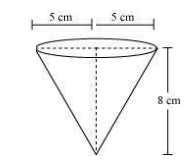Newbie

# A vessel is in the form of an inverted cone. Its height is 8 cm and the radius of its top, which is open, is 5 cm. It is filled with water up to the brim. When lead shots, each of which is a sphere of radius 0.5 cm are dropped into the vessel, one-fourth of the water flows out. Find the number of lead shots dropped in the vessel. Q.5

• 0

Today i am solving the ncert class 10 surface areas and volumes  please give me the simplest solution of exercise 13.1 question number 5 . Find the easiest and best solution of this question . Also find the best formula for this question.A vessel is in the form of an inverted cone. Its height is 8 cm and the radius of its top, which is open, is 5 cm. It is filled with water up to the brim. When lead shots, each of which is a sphere of radius 0.5 cm are dropped into the vessel, one-fourth of the water flows out. Find the number of lead shots dropped in the vessel.

Share

1. Solution:

For the cone,

Radius = 5 cm,

Height = 8 cm

Also,

Radius of sphere = 0.5 cm

The diagram will be likeIt is known that,

Volume of cone = volume of water in the cone

= ⅓πr2h = (200/3)π cm3

Now,

Total volume of water overflown= (¼)×(200/3) π =(50/3)π

Volume of lead shot

= (4/3)πr3

= (1/6) π

Now,

The number of lead shots = Total Volume of Water over flown/ Volume of Lead shot

= (50/3)π/(⅙)π

= (50/3)×6 = 100

• 0